# Show your hand and use Python to analyze house prices

2022-02-02 02:47:04 PI dada

official account ： Youer cottage
author ：Peter
edit ：Peter

Hello everyone , I am a Peter~

This is Kaggle The second column , The title of the competition is ： House Prices - Advanced Regression Techniques. In this paper , You will learn ：

• single 、 Multivariate analysis
• correlation analysis
• Missing and outlier handling
• Dummy variable conversionprimary notebook Address ：www.kaggle.com/pmarcelino/…

## Ranking list

Let's look at the rankings , The first place is really crushing the other players ~ therefore , Today, let's see how great this first place plan is ？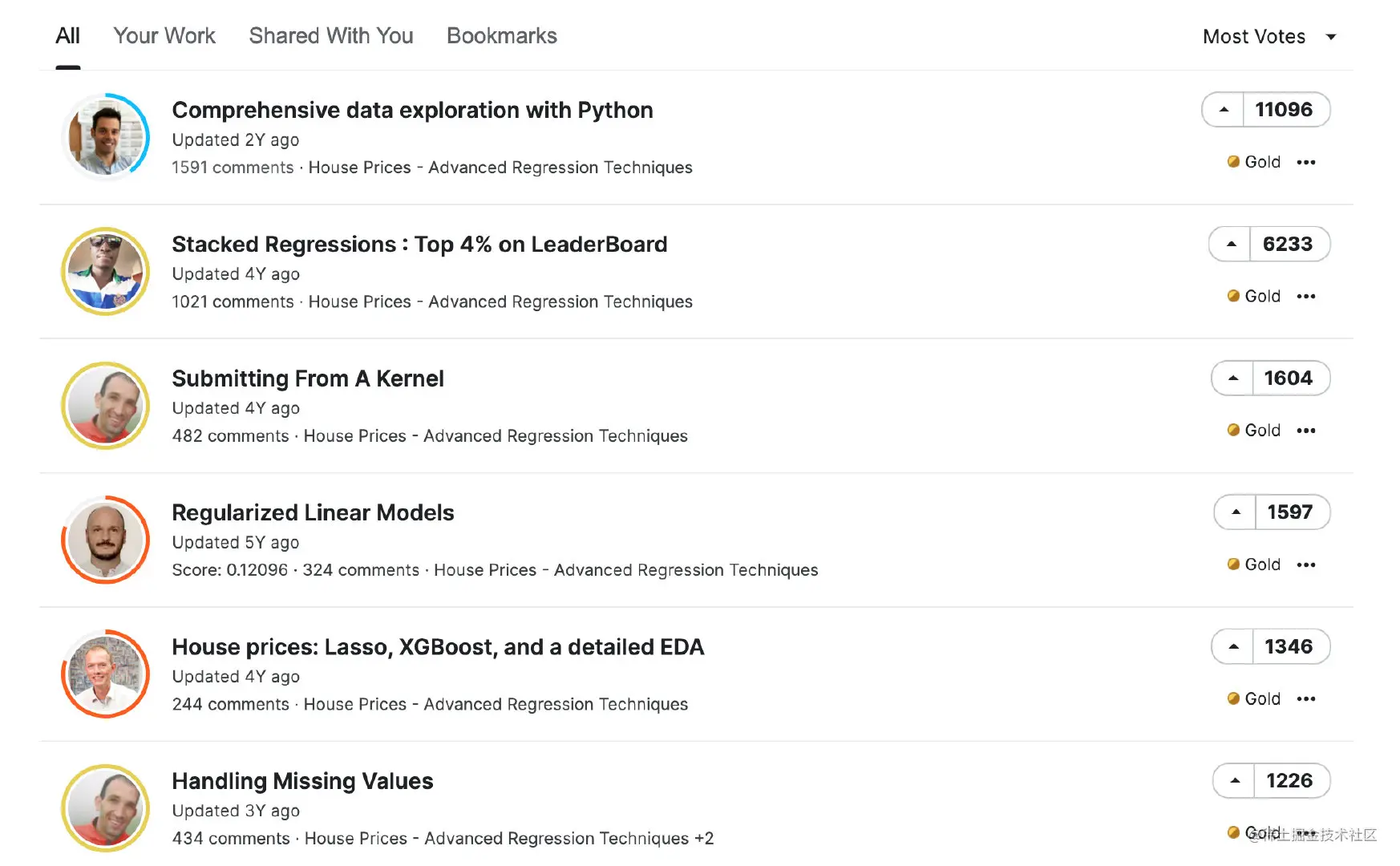## Data is introduced

This Boston house price data set has 4 Copy of the data , Training set train+ Test set test+ Description of the dataset description+ Submit template sample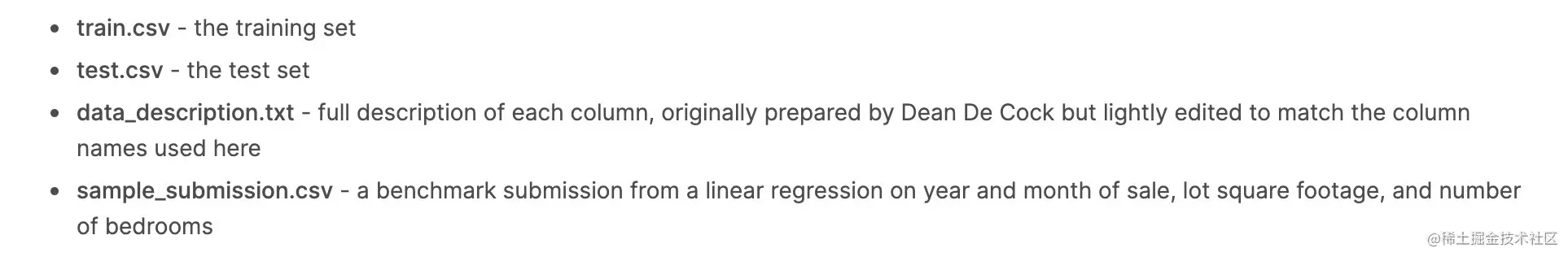The training set includes 81 Features ,1460 Data ; Test set 81 Features ,1459 Data . look down Some properties Introduce ：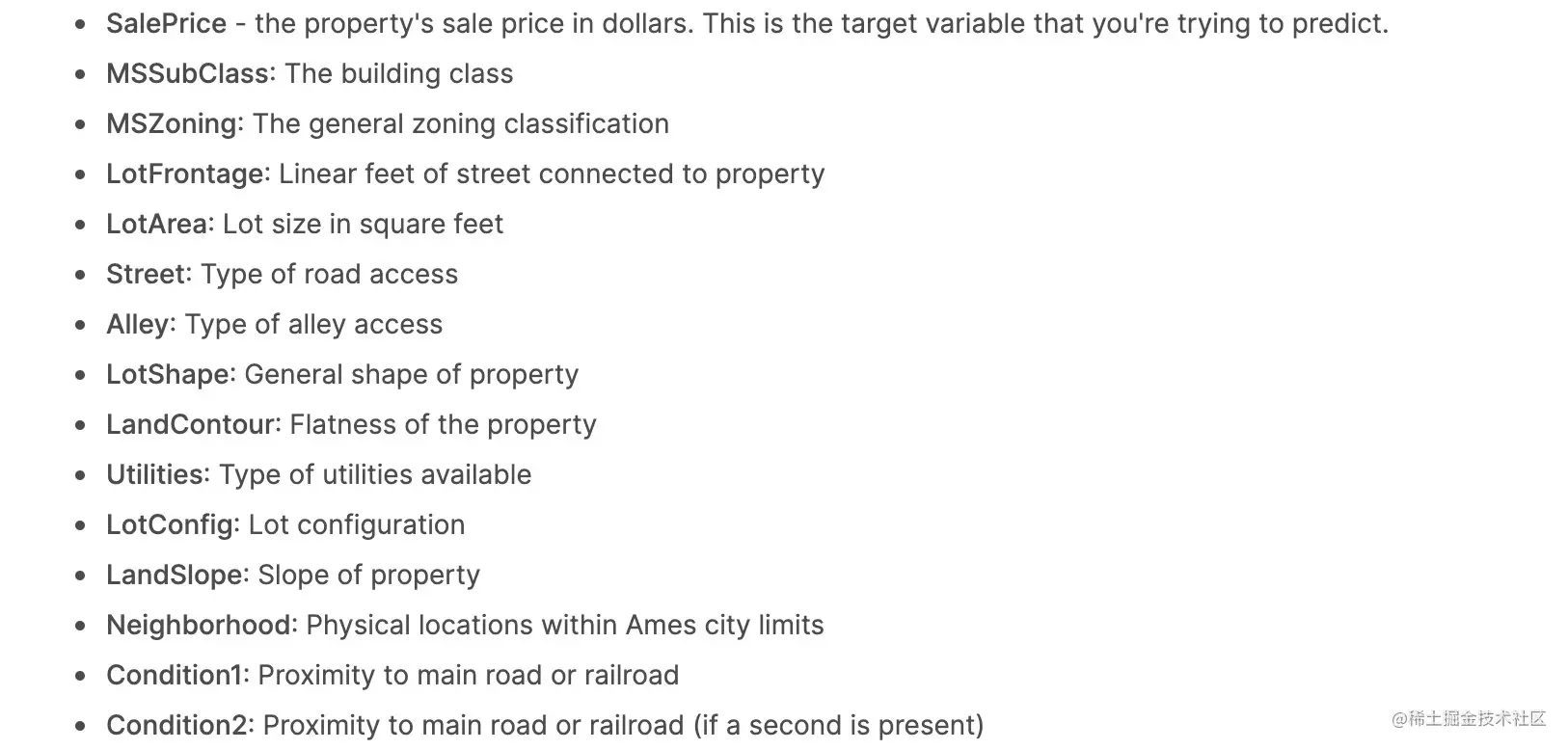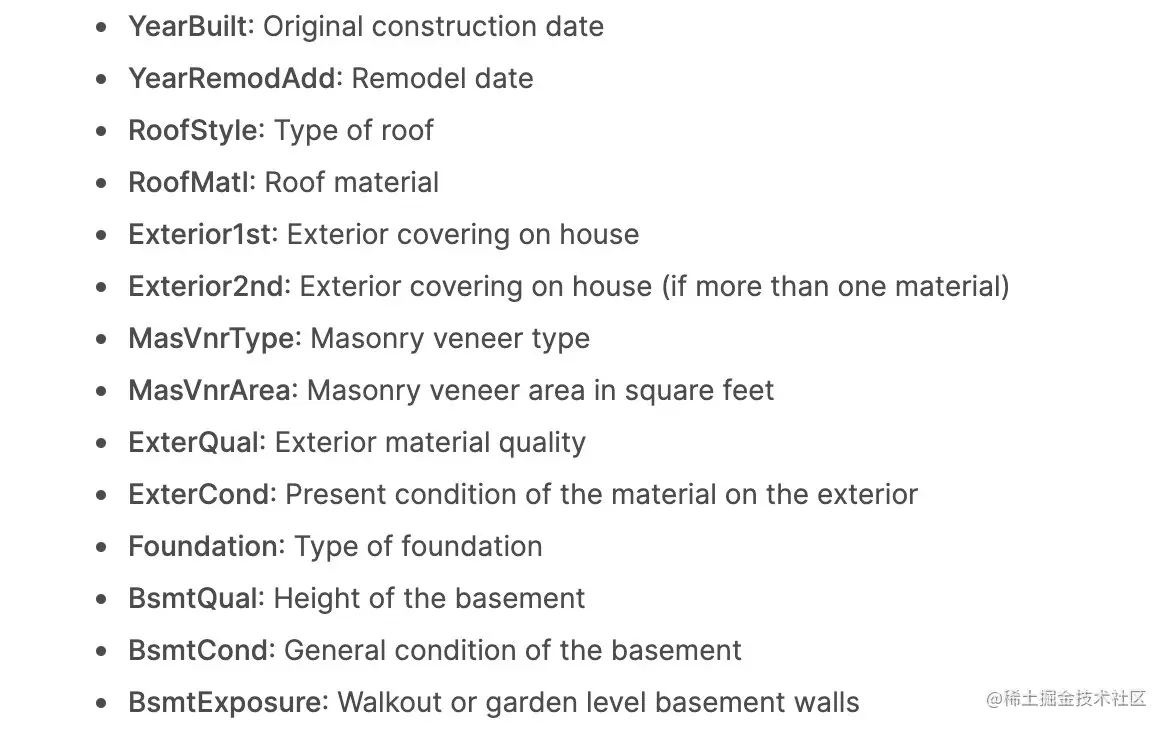## data EDA

Import modules and data , And data exploration ：

### Import library

``````import pandas as pd
import numpy as np
#  Drawing related
import plotly.express as px
import matplotlib.pyplot as plt
import seaborn as sns
plt.style.use("fivethirtyeight")

#  Data modeling
from scipy.stats import norm
from scipy import stats
from sklearn.preprocessing import StandardScaler

#  Warning
import warnings
warnings.filterwarnings('ignore')
%matplotlib inline
Copy code ``````

### Import data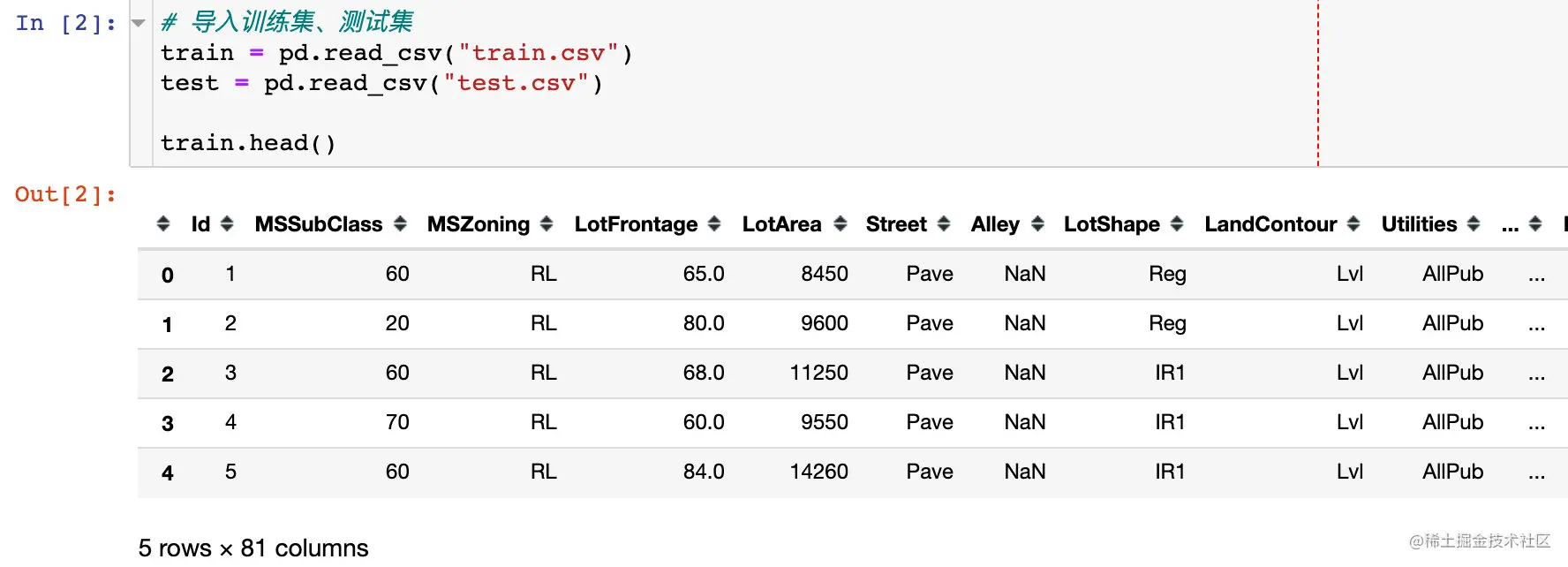### Data and information

The training set as a whole is 1460*81; And many existing fields have missing values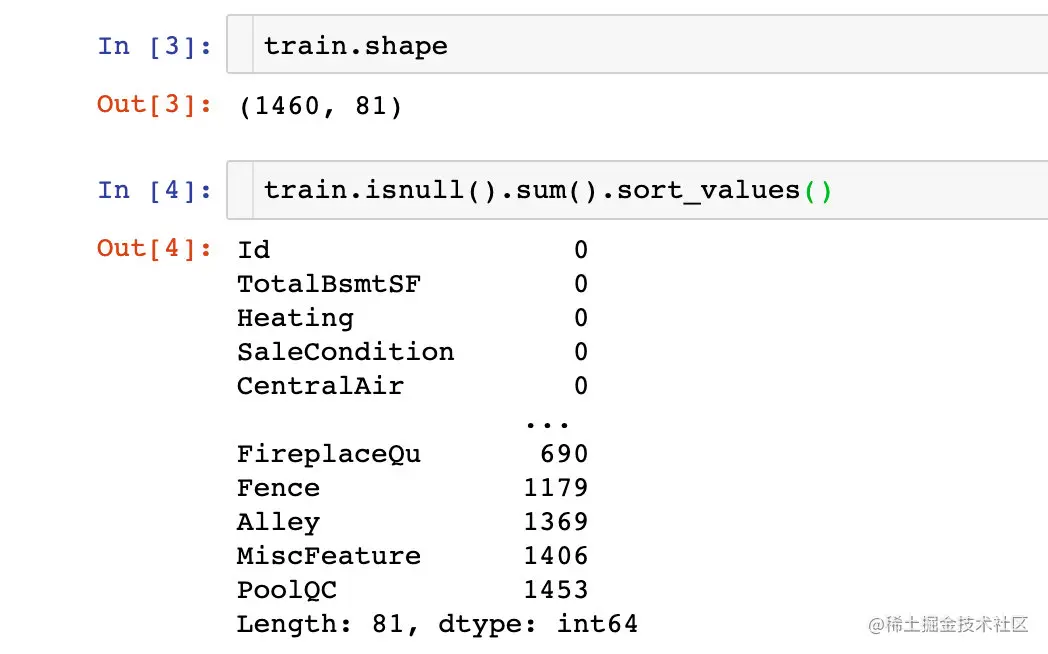Describe statistics ：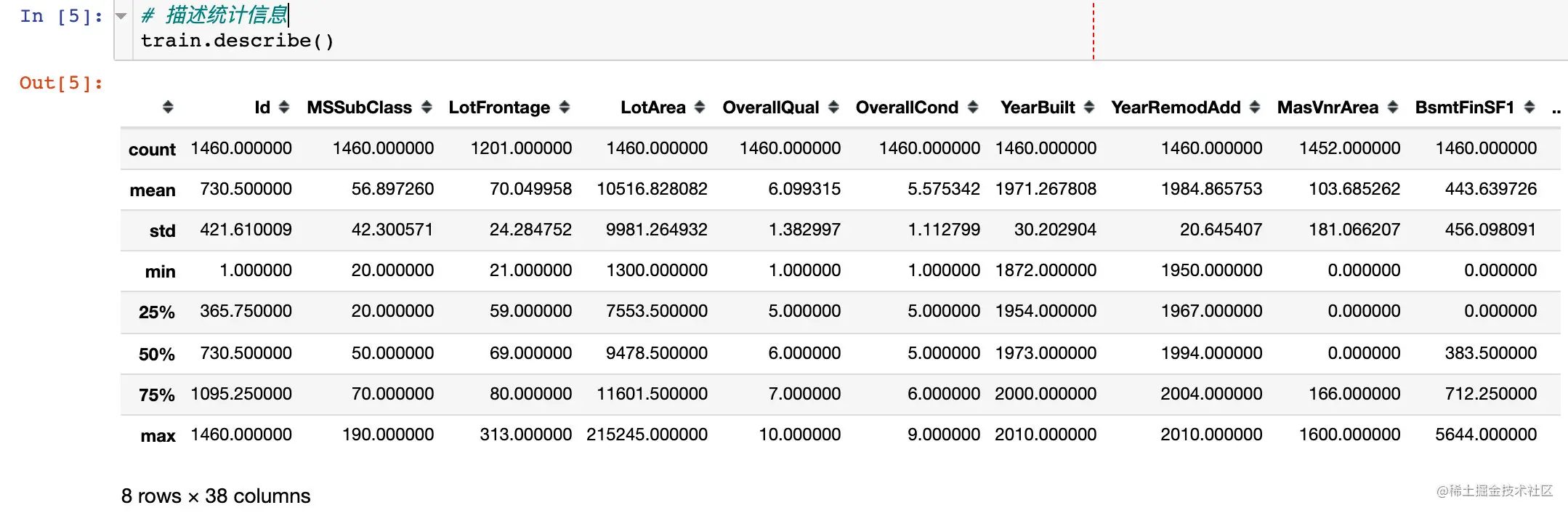## Selling price SalePrice analysis

Maehara notebook In the document , The author has analyzed many of his views on this topic and field , Don't elaborate . The following is the key part ：

### Statistics

Just look at the statistics of this field ：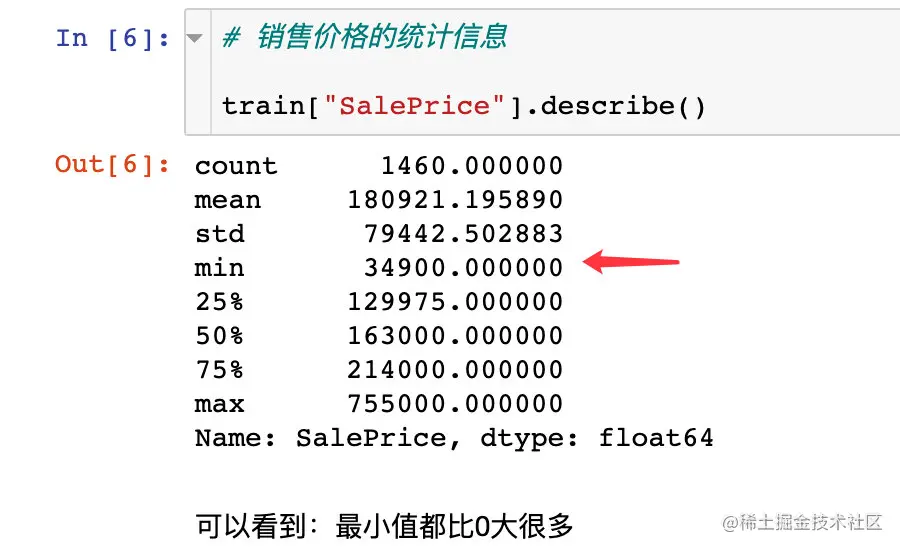The distribution histogram is as follows , We clearly feel ：

• The distribution of prices deviates from the normal distribution
• There is obvious positive skewness
• There are obvious peaks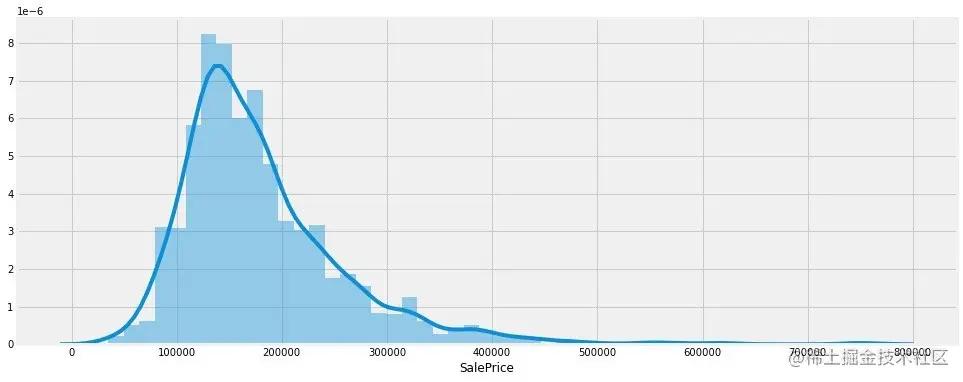### Skewness and kurtosis （skewness and kurtosis）

Knowledge gas station ： Skewness and kurtosis

For a detailed explanation, see the article ：zhuanlan.zhihu.com/p/53184516

• skewness ： Measure the asymmetry of the probability distribution of random face change , It's a measure of the degree of asymmetry relative to the average , By measuring the skewness coefficient , We can determine the degree of asymmetry and direction of data distribution .
• kurtosis ： It is a statistic that studies the steep or smooth distribution of data , By measuring the kurtosis coefficient , We can determine whether the data is steeper or smoother than the normal distribution . The kurtosis is close to 0, The data are normally distributed ; kurtosis >0, High tip distribution ; kurtosis <0, Short and fat distribution

Two distributions of skewness ：

• If it's left , Then the skewness is less than 0
• If it's right , Then the skewness is greater than 0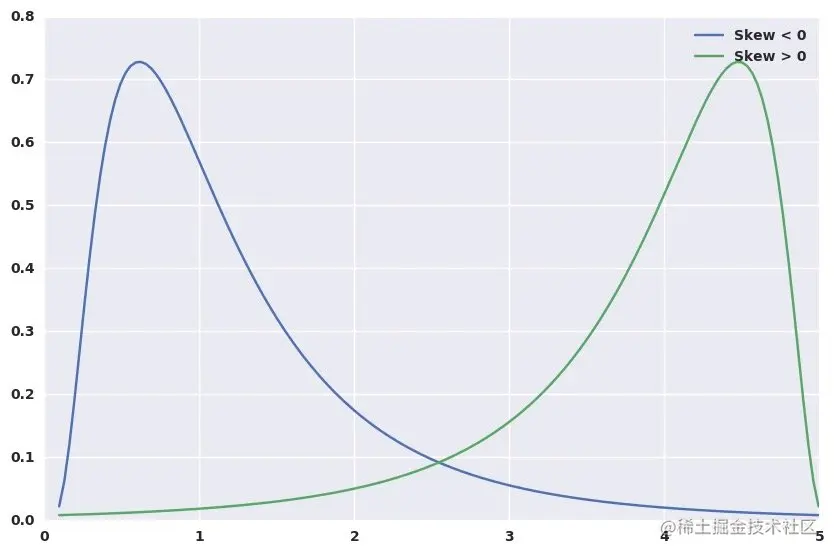Two distributions of kurtosis ：

• If it's tall and thin , Then the kurtosis is greater than 0
• If it's short and fat , Then the kurtosis is less than 0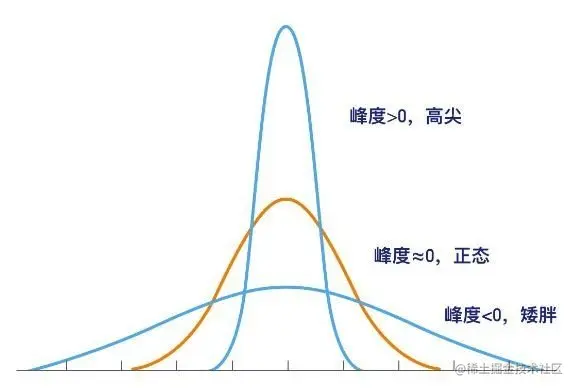``````#  Print the skewness and kurtosis of the sales price

print("Skewness（ skewness ）: %f" % train['SalePrice'].skew())
print("Kurtosis（ kurtosis ）: %f" % train['SalePrice'].kurt())

Skewness（ skewness ）: 1.882876
Kurtosis（ kurtosis ）: 6.536282
Copy code ``````

Skewness and kurtosis are positive , It clearly shows that the data is right biased and high sharp distribution

### SalePrice And numeric fields

First, we examine the relationship between and living area ：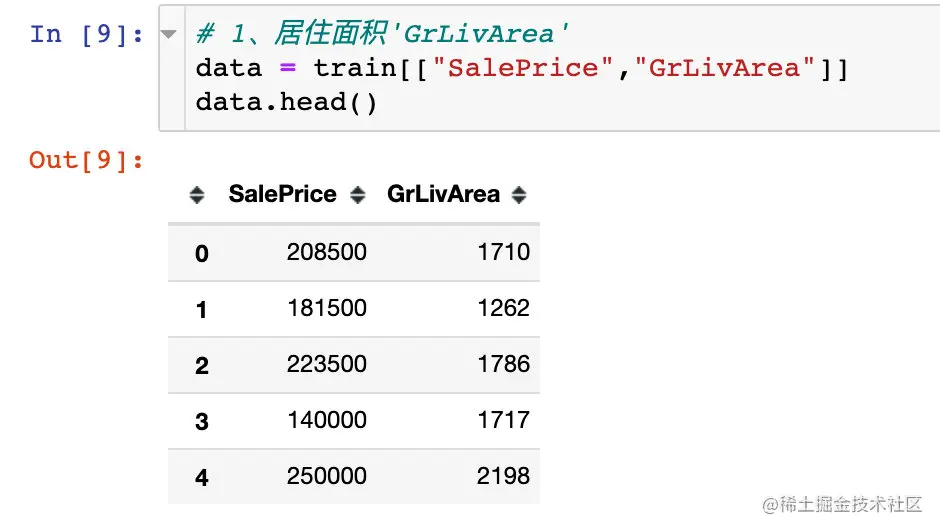``````plt.figure(1,figsize=(12,6))
sns.scatterplot(x="GrLivArea",y="SalePrice",data=data)
plt.show()
Copy code ``````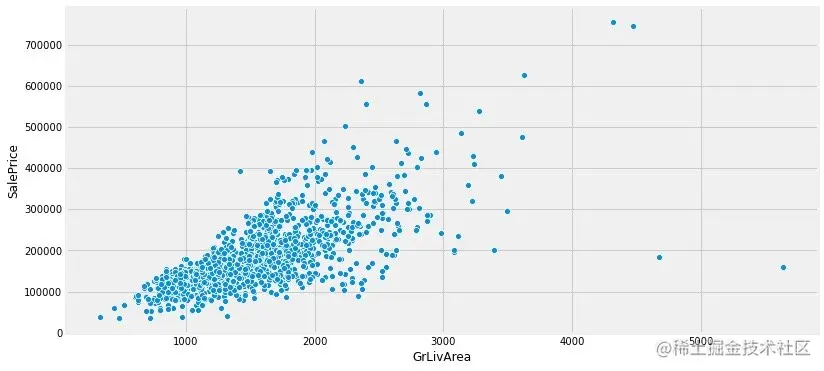``````# plotly edition
px.scatter(data,x="GrLivArea",y="SalePrice",trendline="ols")
Copy code ``````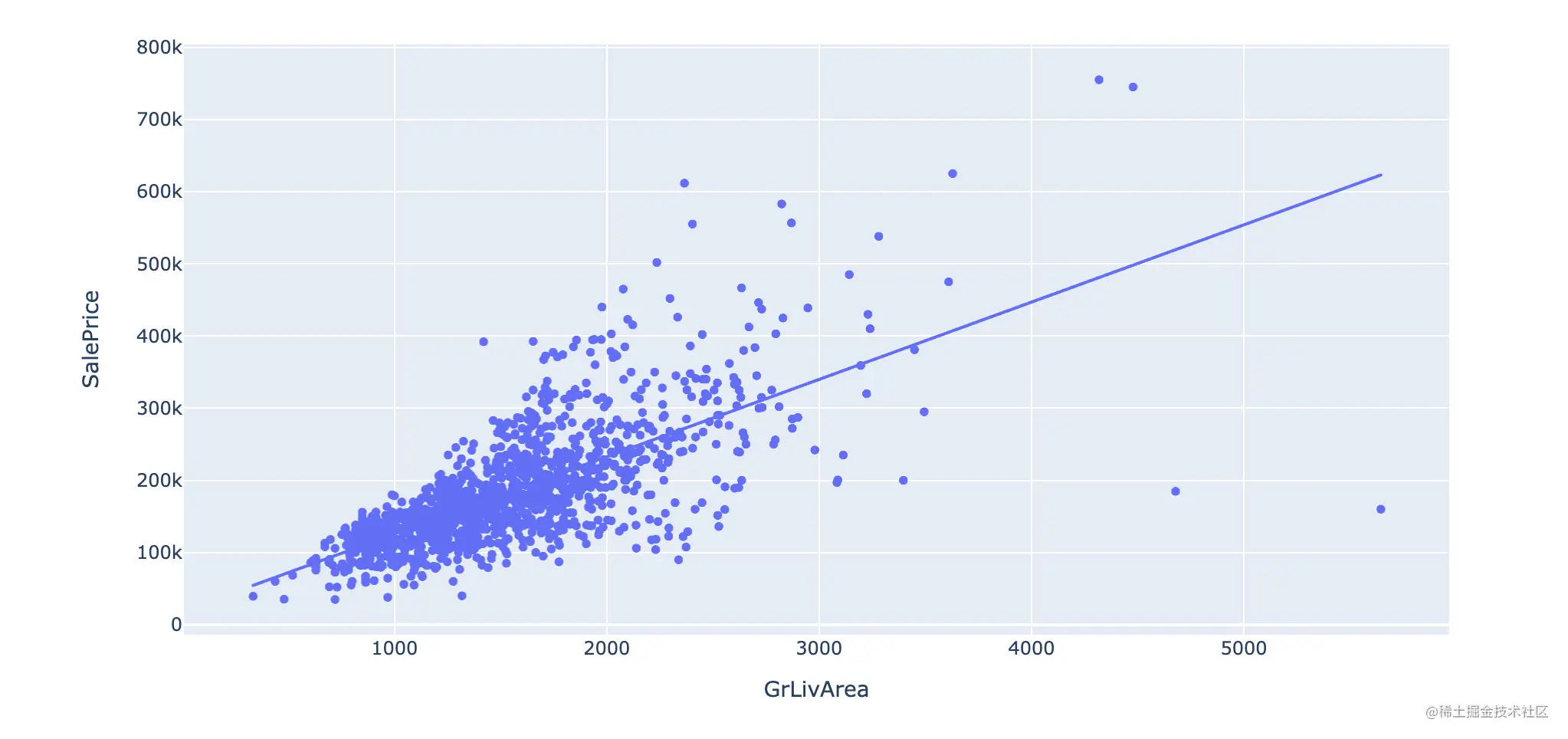#### TotalBsmtSF VS SalePrice

``````# 2、TotalBsmtSF
data = train[["SalePrice","TotalBsmtSF"]]

plt.figure(1,figsize=(12,6))
sns.scatterplot(x="TotalBsmtSF",y="SalePrice",data=data)
plt.show()
Copy code ``````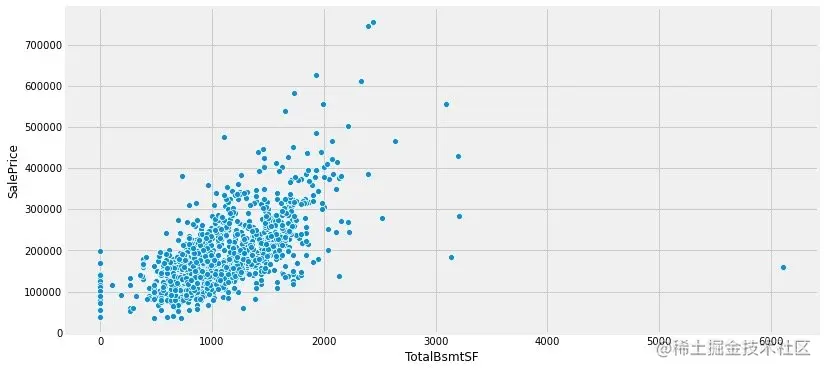Summary ： We can observe that there is a certain linear relationship between these two characteristics and sales price .

### Relationship between price and category field

1、OverallQual VS SalePrice

``````# 1、OverallQual： Overall house quality

#  in total 10 Categories
train["OverallQual"].value_counts()
Copy code ``````
``````5     397
6     374
7     319
8     168
4     116
9      43
3      20
10     18
2       3
1       2
Name: OverallQual, dtype: int64
Copy code ``````
``````data = train[["SalePrice","OverallQual"]]

#  The relationship between the overall quality of the house and the house price
#  Draw a subgraph ：1 No. A
f,ax = plt.subplots(1,figsize=(12,6))
fig = sns.boxplot(x="OverallQual",y="SalePrice",data=data)
# y The scale range of the axis
fig.axis(ymin=0,ymax=800000)
plt.show()
Copy code ``````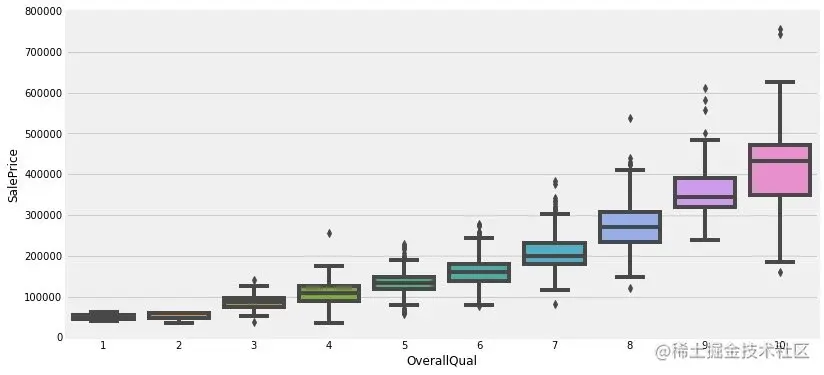2、YearBuilt VS SalePrice

The relationship between the year of construction and the selling price

``````data = train[["SalePrice","YearBuilt"]]

#  Relationship between construction year and house price
f,ax = plt.subplots(1,figsize=(16,8))
fig = sns.boxplot(x="YearBuilt",y="SalePrice",data=data)
# y The scale range of the axis
fig.axis(ymin=0,ymax=800000)
plt.show()
Copy code ``````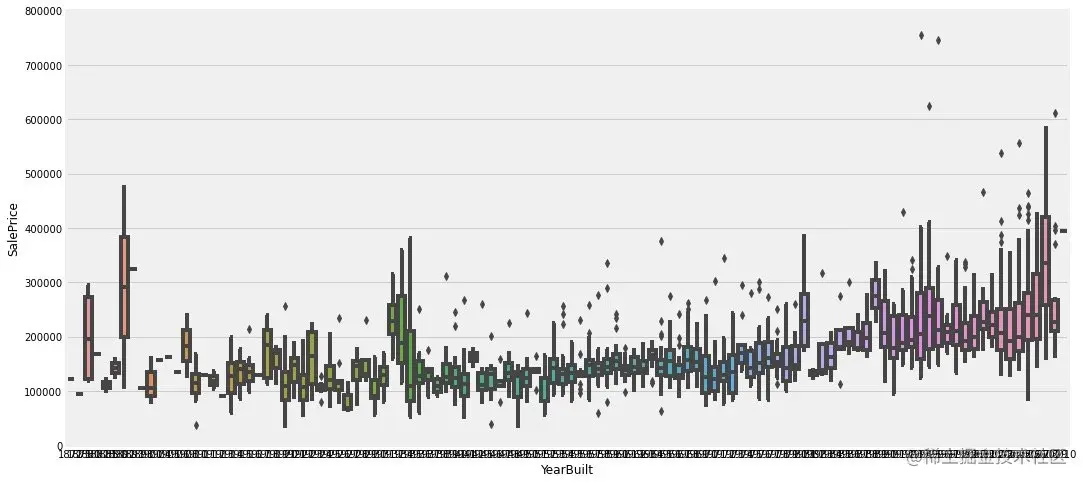Summary ： The sales price has a strong relationship with the overall quality of the house ; But it has little to do with the year of construction . But in the actual process of buying a house , We still care about the year

### Summary

A summary of the above analysis ：

1. Ground living area (GrLivArea)、 Basement area (GrLivArea) And selling price SalePrice All show positive linear correlation
2. The overall quality of the house (OverallQual) And year of construction (YearBuilt) It also seems to be linearly related to the sales price . Common sense , The better the overall quality , The more expensive it is

## correlation analysis

In order to explore the relationship between many attributes , Carry out the following analysis ：

• Correlation between two attributes （ Heat map ）
• Selling price saleprice Relationship with other attributes （ Heat map ）
• The relationship between the most relevant attributes （ Scatter plot ）

### Overall relevance

Analyze the correlation of each two attributes , And draw a thermal diagram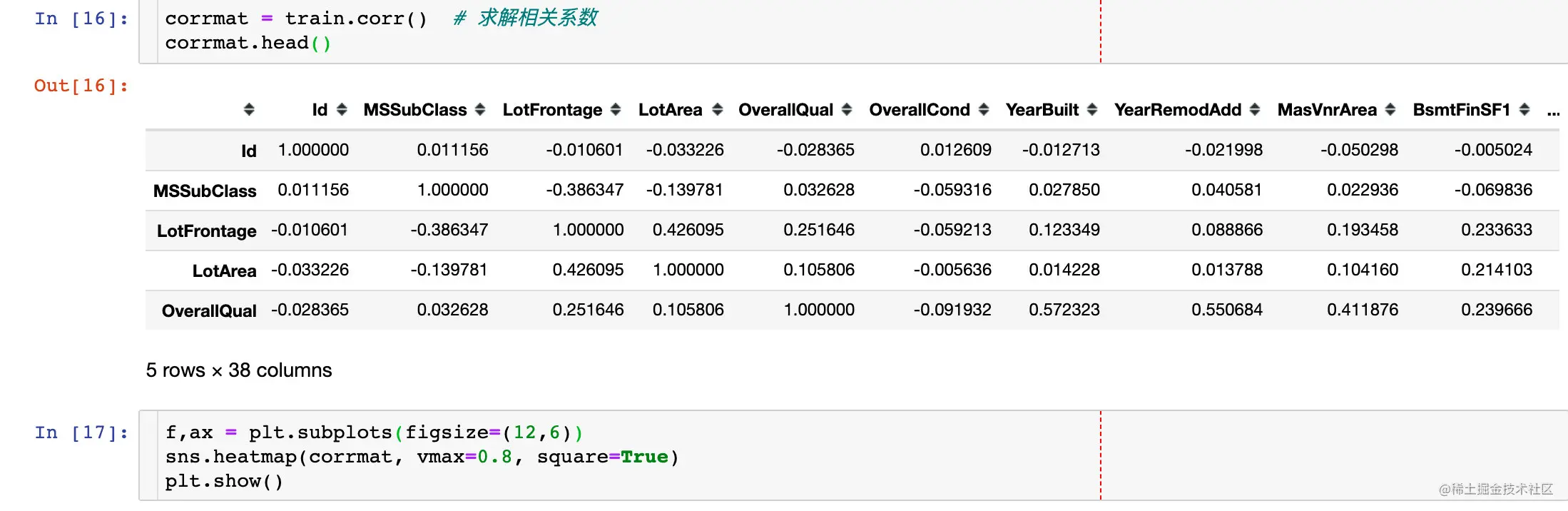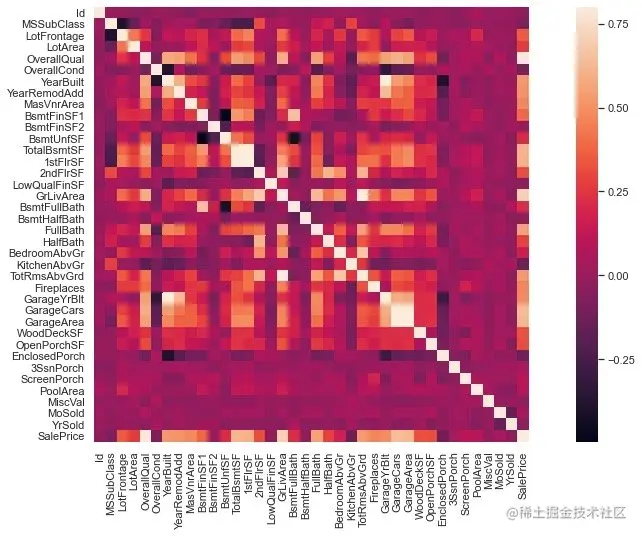There are two noteworthy points in the figure above ：

• TotalBsmtSF and 1stFlrSF
• GarageCar and GarageArea

Both sets of variables are strongly correlated , Our subsequent analysis only takes one of them

### Scale the correlation matrix （ Selling price saleprice）

from The heat map above Select and SalePrice The most relevant former 10 A feature to draw a thermal map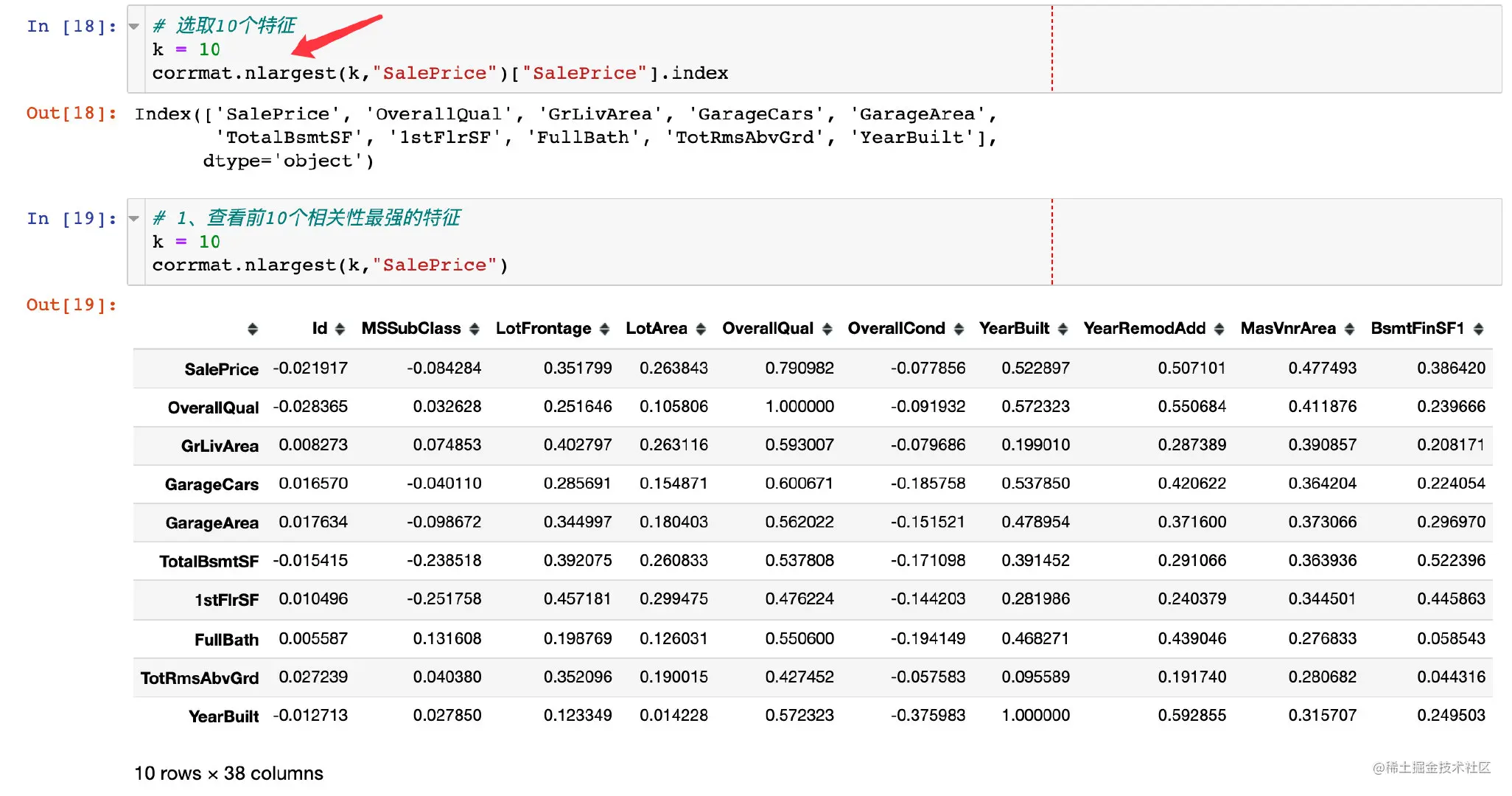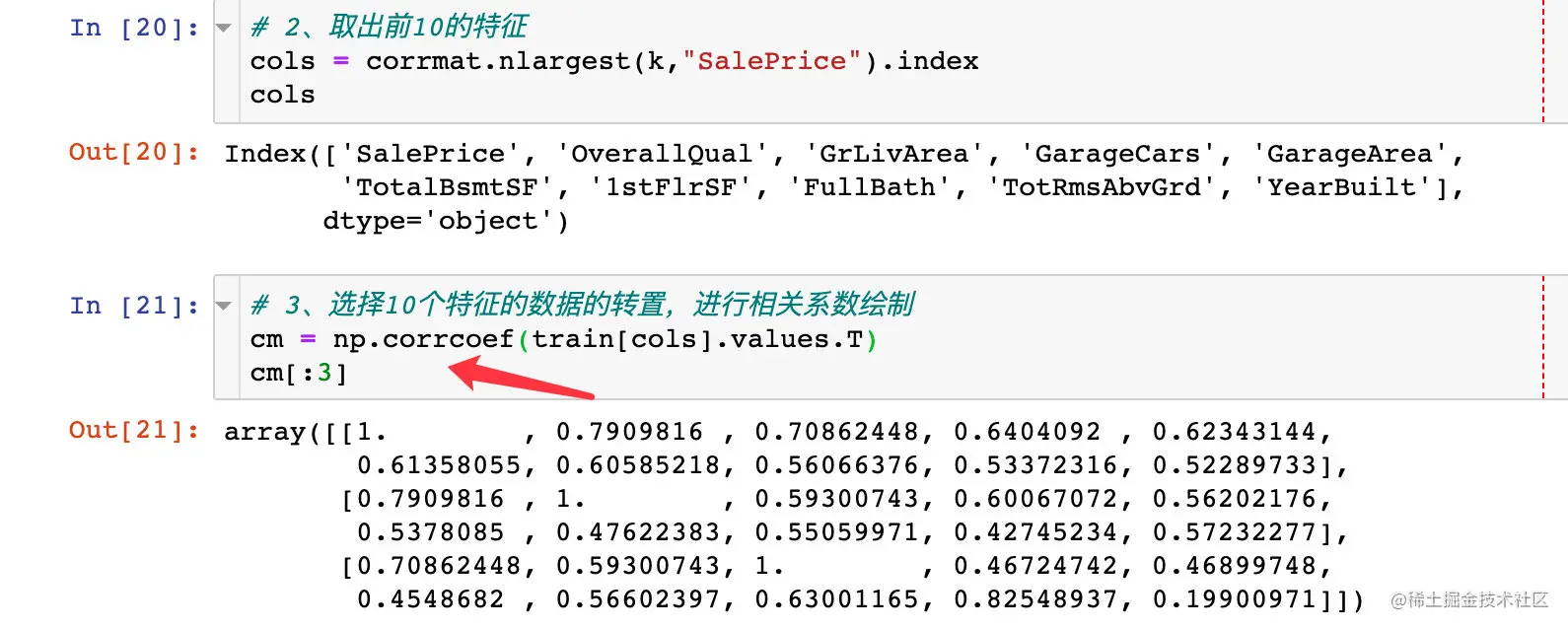``````sns.set(font_scale=1.25)
hm = sns.heatmap(
cm,  #  The drawing data
cbar=True,  #  Whether to use the color bar as a legend , Default True
annot=True,  #  Display value
square=True,  #  Whether to make each unit of the thermodynamic diagram square , The default is False
fmt='.2f',  #  Keep two decimal places
annot_kws={'size':10},
xticklabels=cols.values, # xy Axis settings
yticklabels=cols.values)

plt.show()
Copy code ``````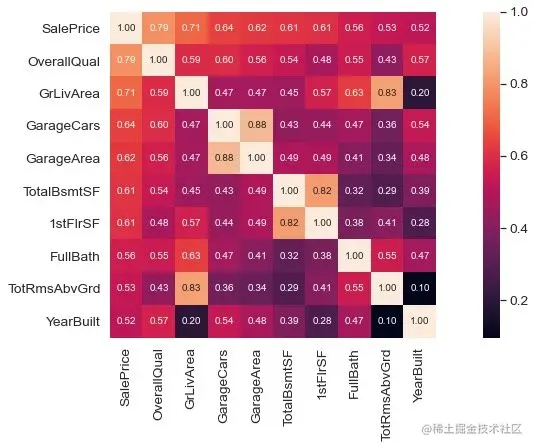### Summary 1

By scaling the heat map above , We can draw the following conclusion ：

• 'OverallQual', 'GrLivArea' and 'TotalBsmtSF' It's true and 'SalePrice' Show strong correlation
• 'GarageCars' and 'GarageArea' It is also two characteristics with strong correlation ; And they all appear at the same time , Follow up selection GarageCars Analyze
• Construction life 'YearBuilt' relatively speaking , The correlation is relatively low

### Variable discrete graph

Set the selling price SalePrice Together with several highly correlated features , Draw variable discrete diagram

``````sns.set()
#  Variables to be analyzed
cols = ['SalePrice', 'OverallQual', 'GrLivArea', 'GarageCars', 'TotalBsmtSF', 'FullBath', 'YearBuilt']
sns.pairplot(train[cols],size=2.5)
plt.show()
Copy code ``````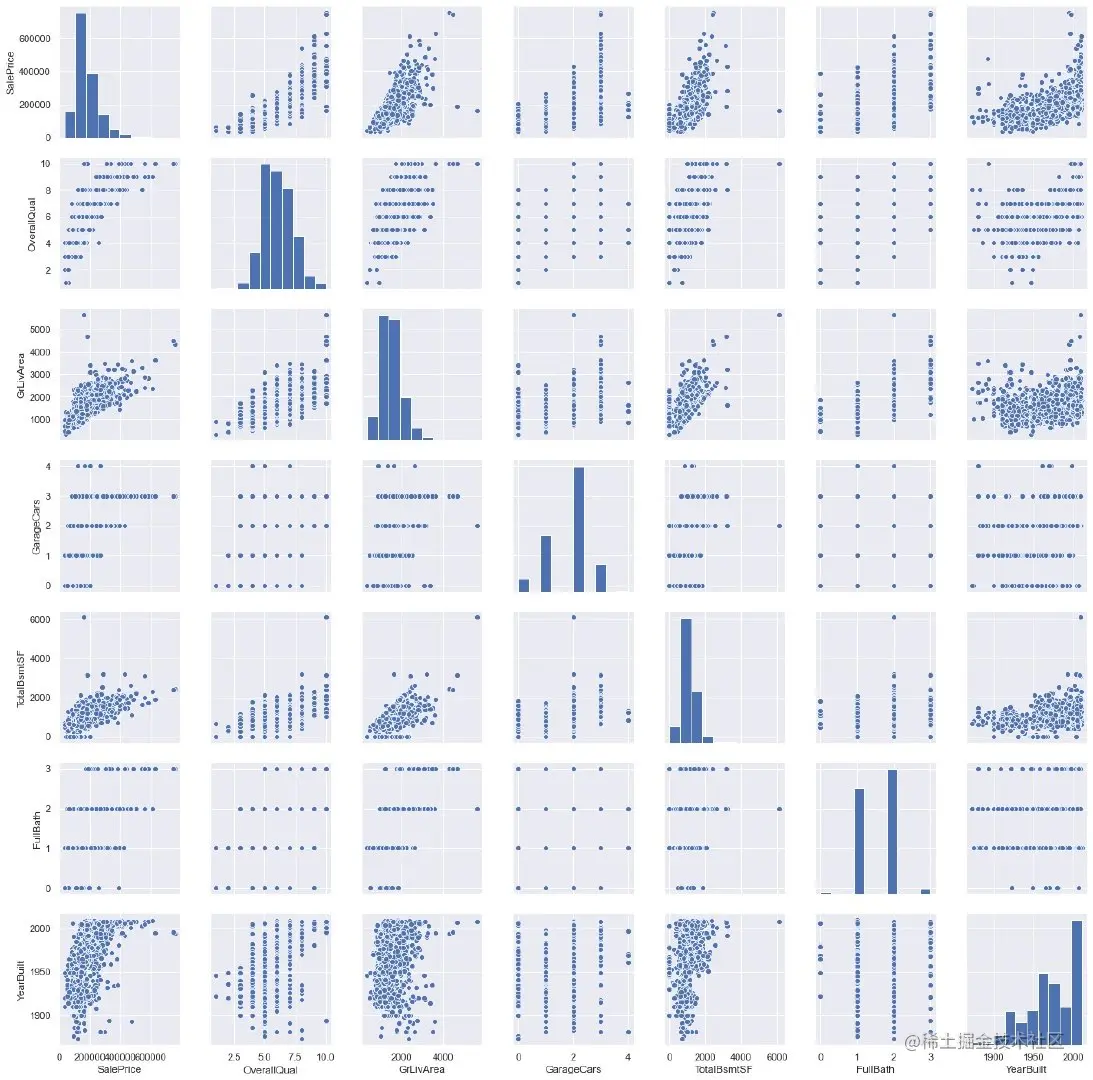### Summary 2

In the diagonal direction is the histogram of the variable , Explanatory variables and explained variables SalePrice, Others are scatter charts .

If the graph shows scattered points of straight lines or horizontal lines , It means that the variable is discrete , For example 1 That's ok 4 Column variables ,y The axis is SalePrice,x The axis is YearBuilt, Straight line description YearBuilt Is discrete

## Missing value processing

For missing values , It mainly discusses two points ：

• What is the distribution of missing values ？
• Missing values are random ？ There is also a certain law

### Proportion of missing values

1、 Check the missing value of each field

``````#  Number of missing values per field ： Descending
total = train.isnull().sum().sort_values(ascending=False)
Copy code ``````
``````PoolQC         1453
MiscFeature    1406
Alley          1369
Fence          1179
FireplaceQu     690
dtype: int64
Copy code ``````

2、 Convert to percentage

``````#  Missing value for each field  /  total
percent = (train.isnull().sum() / train.isnull().count()).sort_values(ascending=False)
Copy code ``````
``````PoolQC         0.995205
MiscFeature    0.963014
Alley          0.937671
Fence          0.807534
FireplaceQu    0.472603
dtype: float64
Copy code ``````

3、 Data merging , Overall missing values ：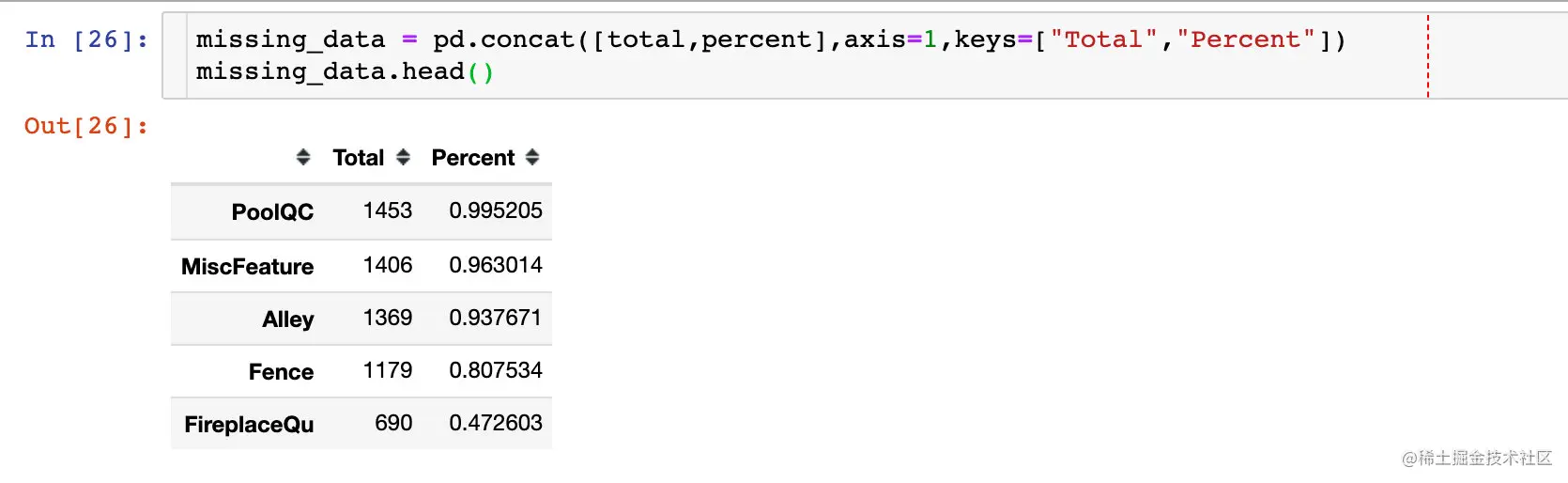### Delete missing value

The original text analyzes a lot , The final conclusion ：

In summary, to handle missing data,

1、we'll delete all the variables with missing data, except the variable 'Electrical'.

2、In 'Electrical' we'll just delete the observation with missing data.

``````#  step 1： Fields to be deleted
missing_data[missing_data["Total"] > 1].index
Copy code ``````
``````Index(['PoolQC', 'MiscFeature', 'Alley', 'Fence', 'FireplaceQu', 'LotFrontage',
'GarageYrBlt', 'GarageCond', 'GarageType', 'GarageFinish', 'GarageQual',
'BsmtFinType2', 'BsmtExposure', 'BsmtQual', 'BsmtCond', 'BsmtFinType1',
'MasVnrArea', 'MasVnrType'],
dtype='object')
Copy code ``````
``````#  First step
train = train.drop(missing_data[missing_data["Total"] > 1].index,1)
#  The second step
train = train.drop(train.loc[train["Electrical"].isnull()].index)
Copy code ``````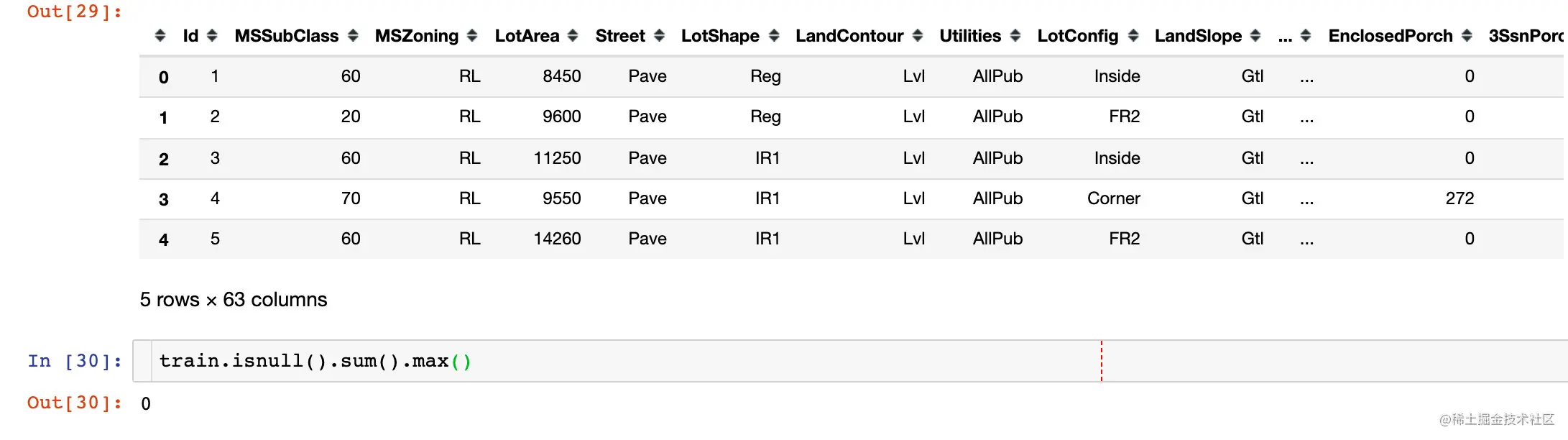## outliers out liars

### Find outliers

``````##  Data standardization standardizing data
# np.newaxis  Add data dimension , One dimension becomes two dimensions
saleprice_scaled = StandardScaler().fit_transform(train["SalePrice"][:,np.newaxis])
saleprice_scaled[:5]
Copy code ``````
``````array([[ 0.34704187],
[ 0.0071701 ],
[ 0.53585953],
[-0.5152254 ],
[ 0.86943738]])
Copy code ``````
``````#  See the former 10 And finally 10 A data
# argsort： What is returned is the index value ; The default is ascending , The smallest is at the front , The biggest is at the end

low_range = saleprice_scaled[saleprice_scaled[:,0].argsort()][:10]

high_range = saleprice_scaled[saleprice_scaled[:,0].argsort()][-10:]

print(low_range)
print('----------')
print(high_range)
Copy code ``````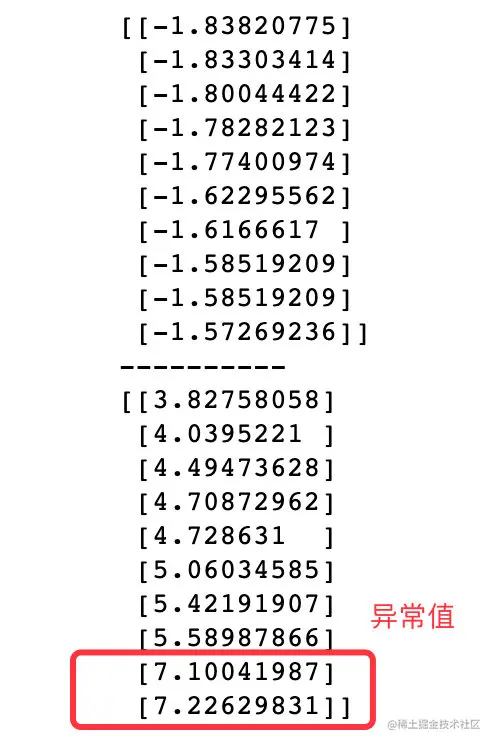### Summary 3

• low_range near , And leave 0 It's closer
• high_range leave 0 Far away ; And 7+ The data should be outliers

### Univariate analysis 1

``````data = train[["SalePrice","GrLivArea"]]
data.plot.scatter(x="GrLivArea",y="SalePrice",ylim=(0,800000))
plt.show()
Copy code ``````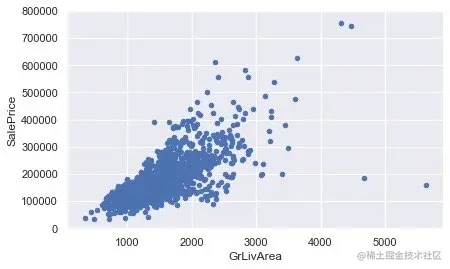The obvious , Two variables （ attribute ） There is a linear relationship

### Delete outliers

Specify the method to delete a field as a specific value ：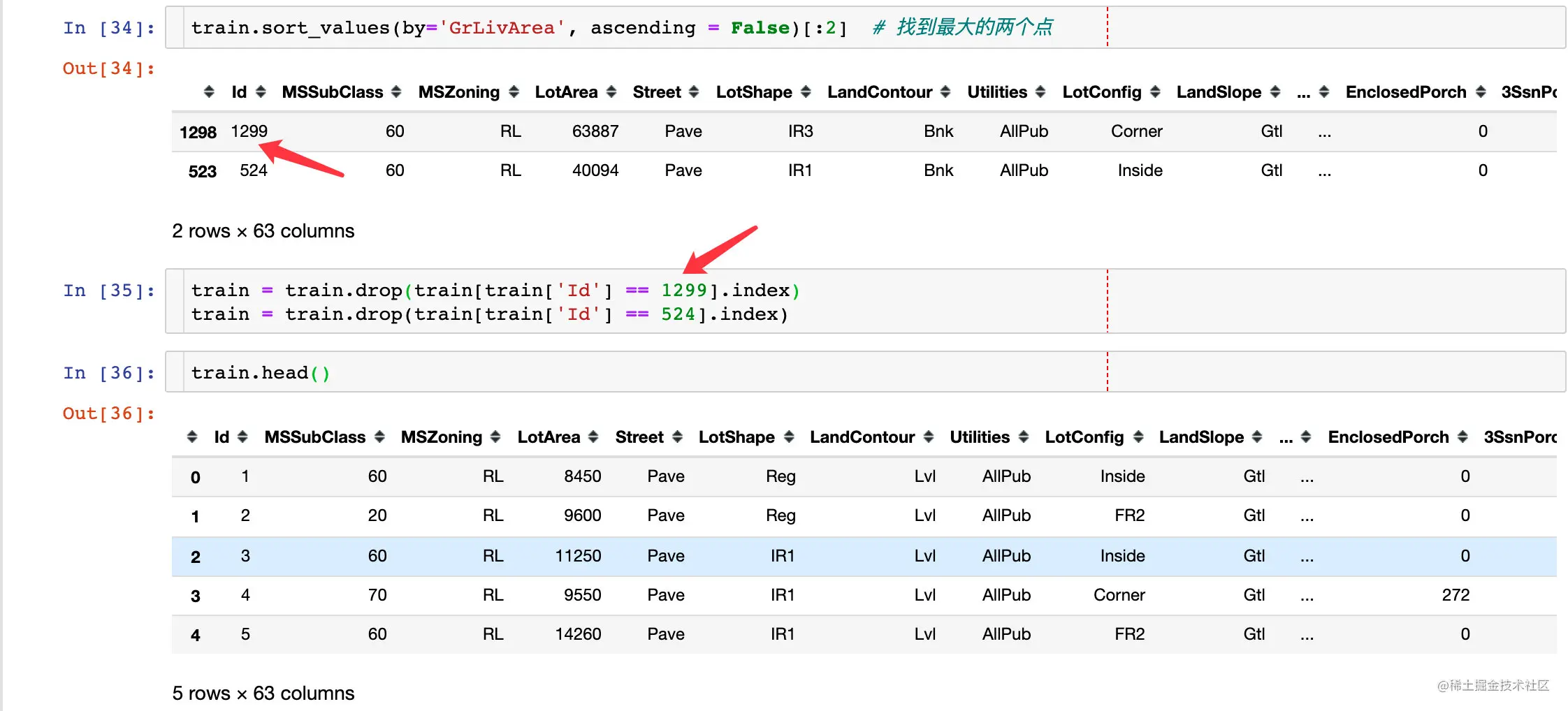### Univariate analysis 2

``````data = train[["SalePrice","TotalBsmtSF"]]   #  Two variables to be analyzed
data.plot.scatter(x="TotalBsmtSF",y="SalePrice",ylim=(0,800000))
plt.show()
Copy code ``````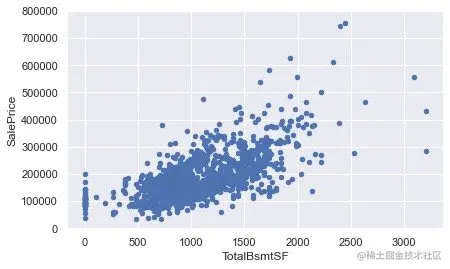## In depth understanding of SalePrice

This paper mainly studies the sales price from the following aspects ：

• Normality： normalization
• Homoscedasticity： homoscedasticity
• Linearity： Linear trait
• Absence of correlated errors： Correlation error

### Normality normalization （SalePrice）

``````sns.distplot(train["SalePrice"],fit=norm)
fig = plt.figure()
res = stats.probplot(train["SalePrice"], plot=plt)
Copy code ``````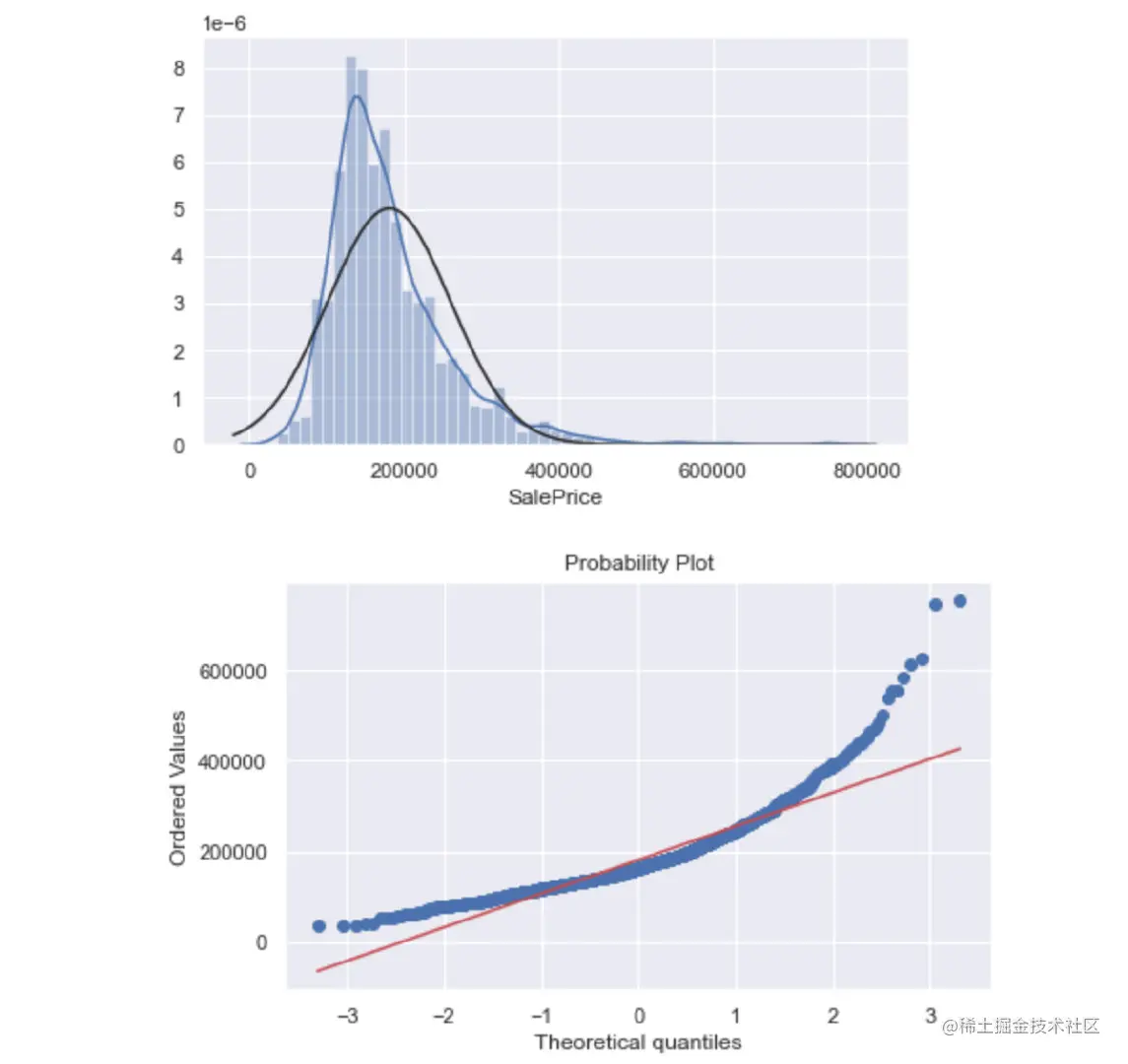We found that ： The selling price is not normally distributed , There is a right deviation ; At the same time, it does not follow the law of logarithmic change .

To solve this problem ： Implement logarithmic transformation

``````##  Logarithmic transformation
train["SalePrice"] = np.log(train["SalePrice"])

sns.distplot(train["SalePrice"],fit=norm)
fig = plt.figure()
res = stats.probplot(train["SalePrice"], plot=plt)
Copy code ``````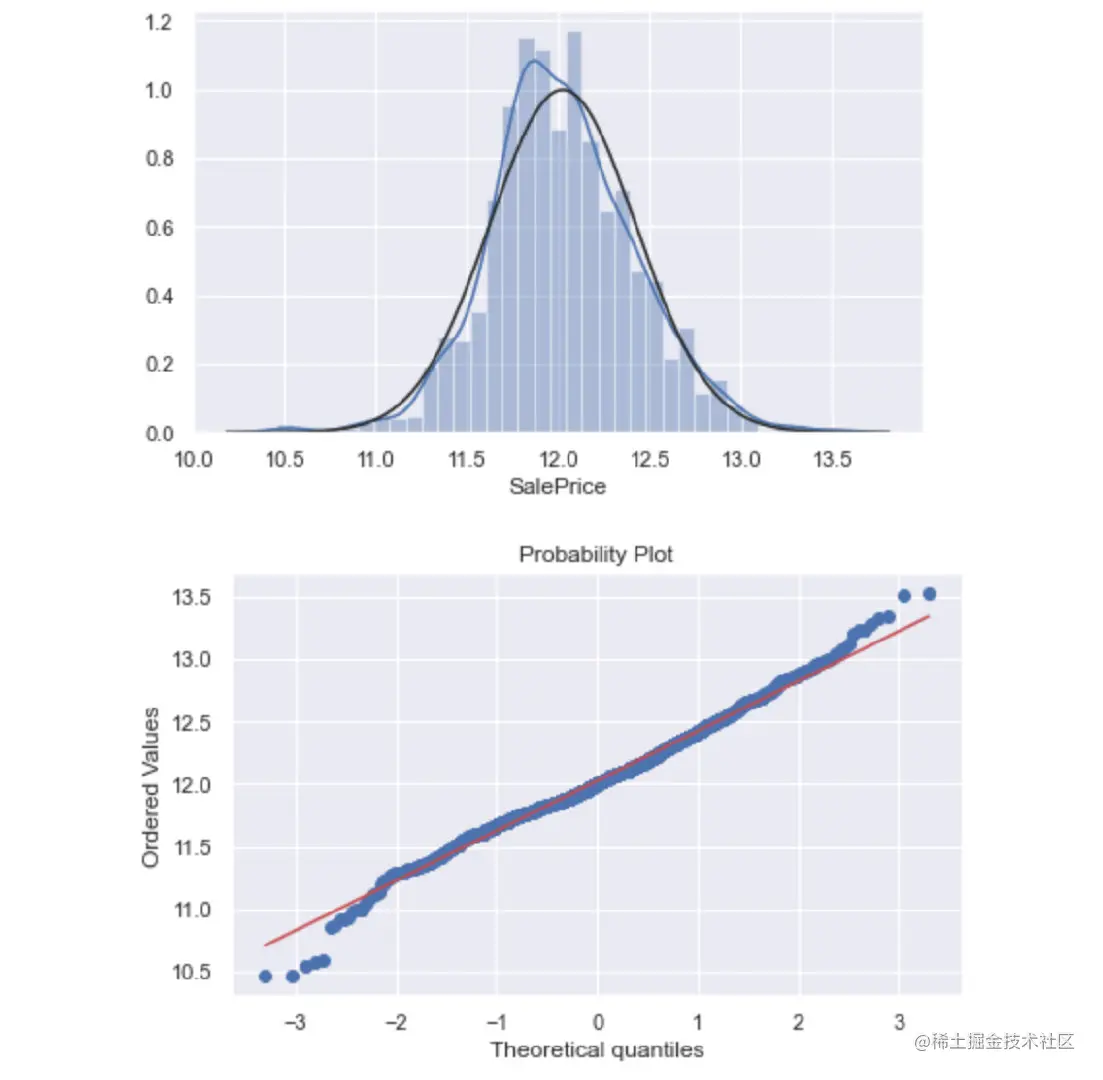After the implementation of logarithmic transformation, the effect is much better

### Normality- normalization （GrLivArea）

``````sns.distplot(train["GrLivArea"],fit=norm)
fig = plt.figure()
res = stats.probplot(train["GrLivArea"], plot=plt)
Copy code ``````

Effect before logarithmic transformation ：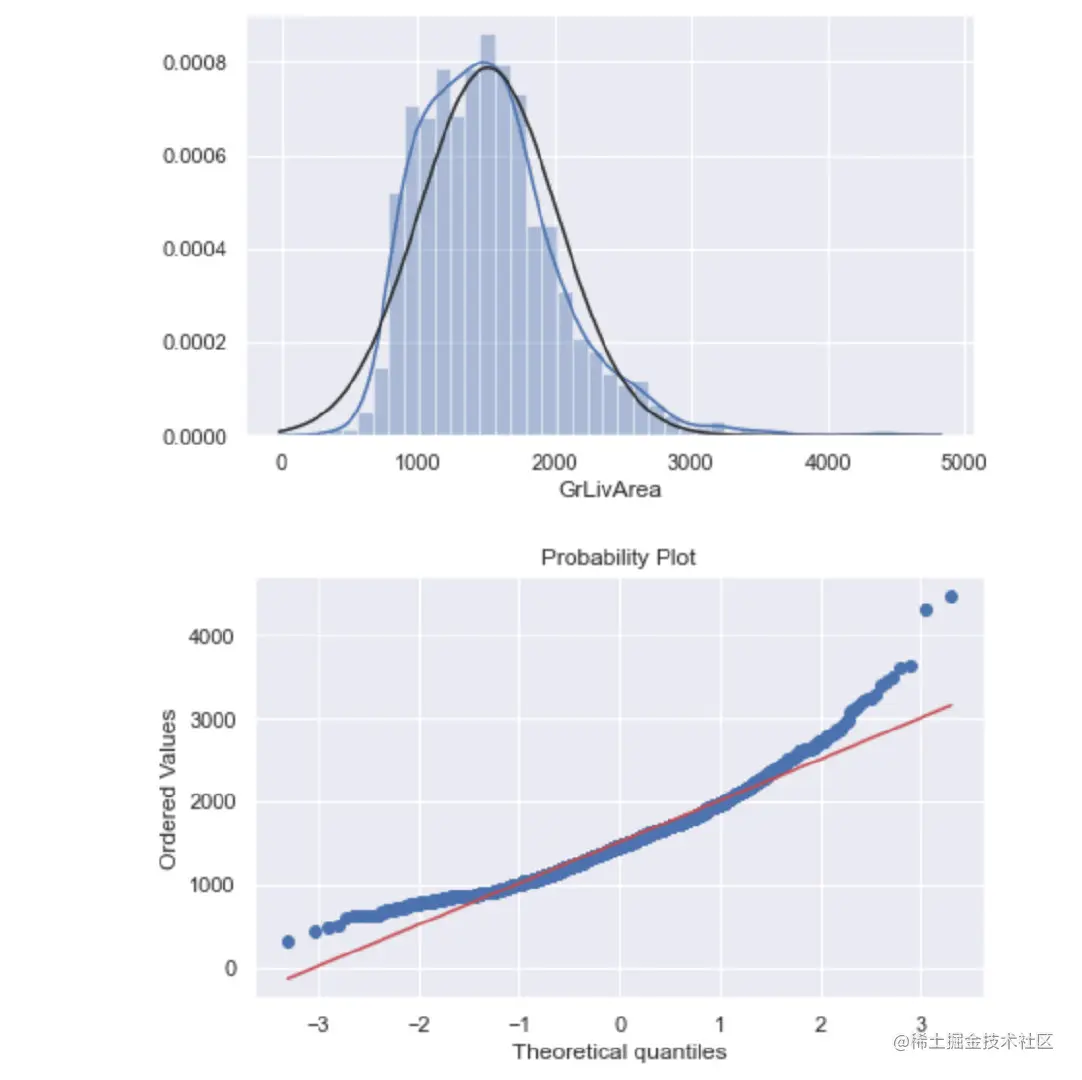Perform logarithmic transformation and effect ：

``````#  Perform the same logarithmic operation
train["GrLivArea"] = np.log(train["GrLivArea"])

sns.distplot(train["GrLivArea"],fit=norm)
fig = plt.figure()
res = stats.probplot(train["GrLivArea"], plot=plt)
Copy code ``````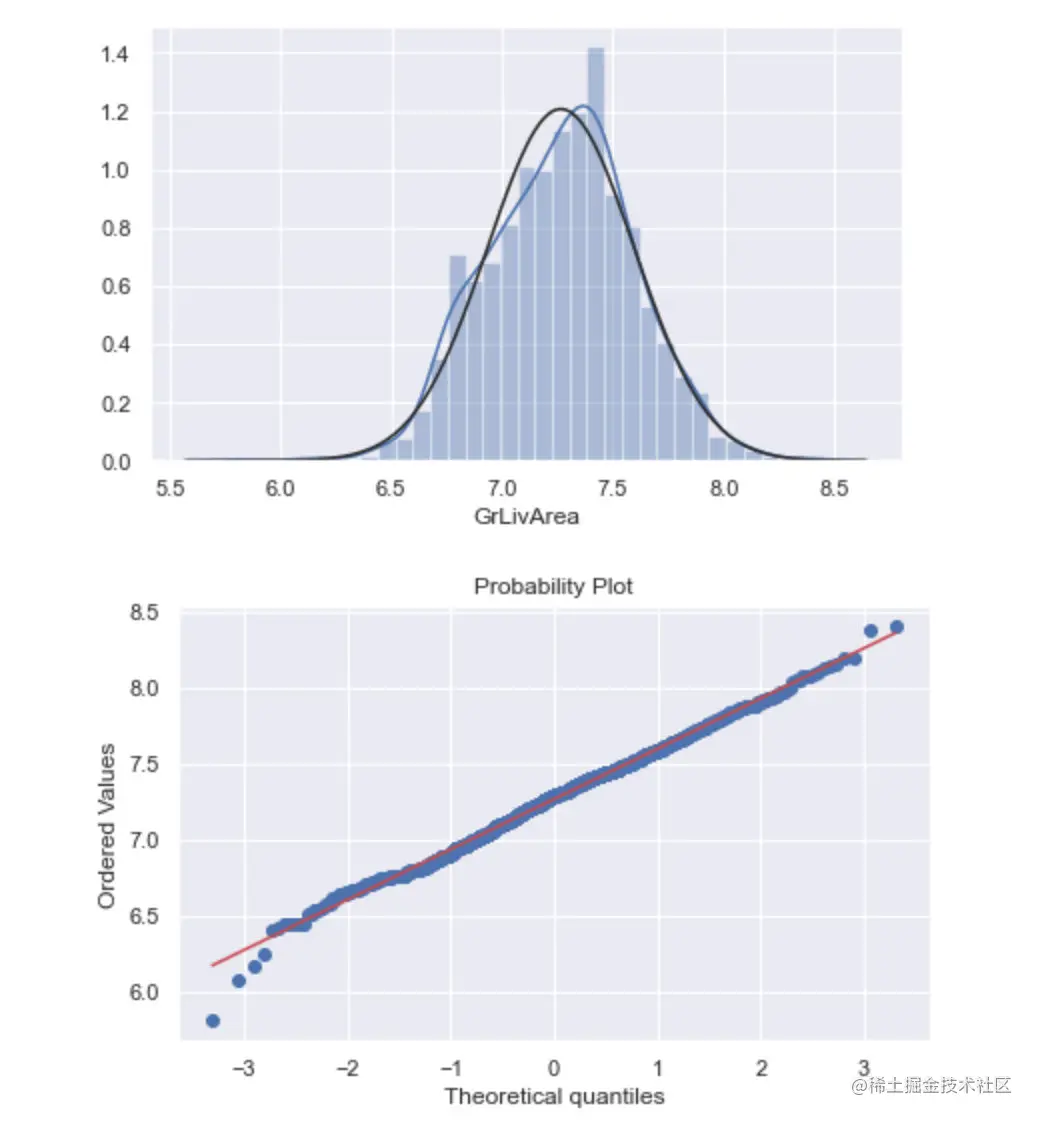### Normality- normalization （TotalBsmtSF）

``````sns.distplot(train["TotalBsmtSF"],fit=norm)
fig = plt.figure()
res = stats.probplot(train["TotalBsmtSF"], plot=plt)
Copy code ``````

Effect before treatment ：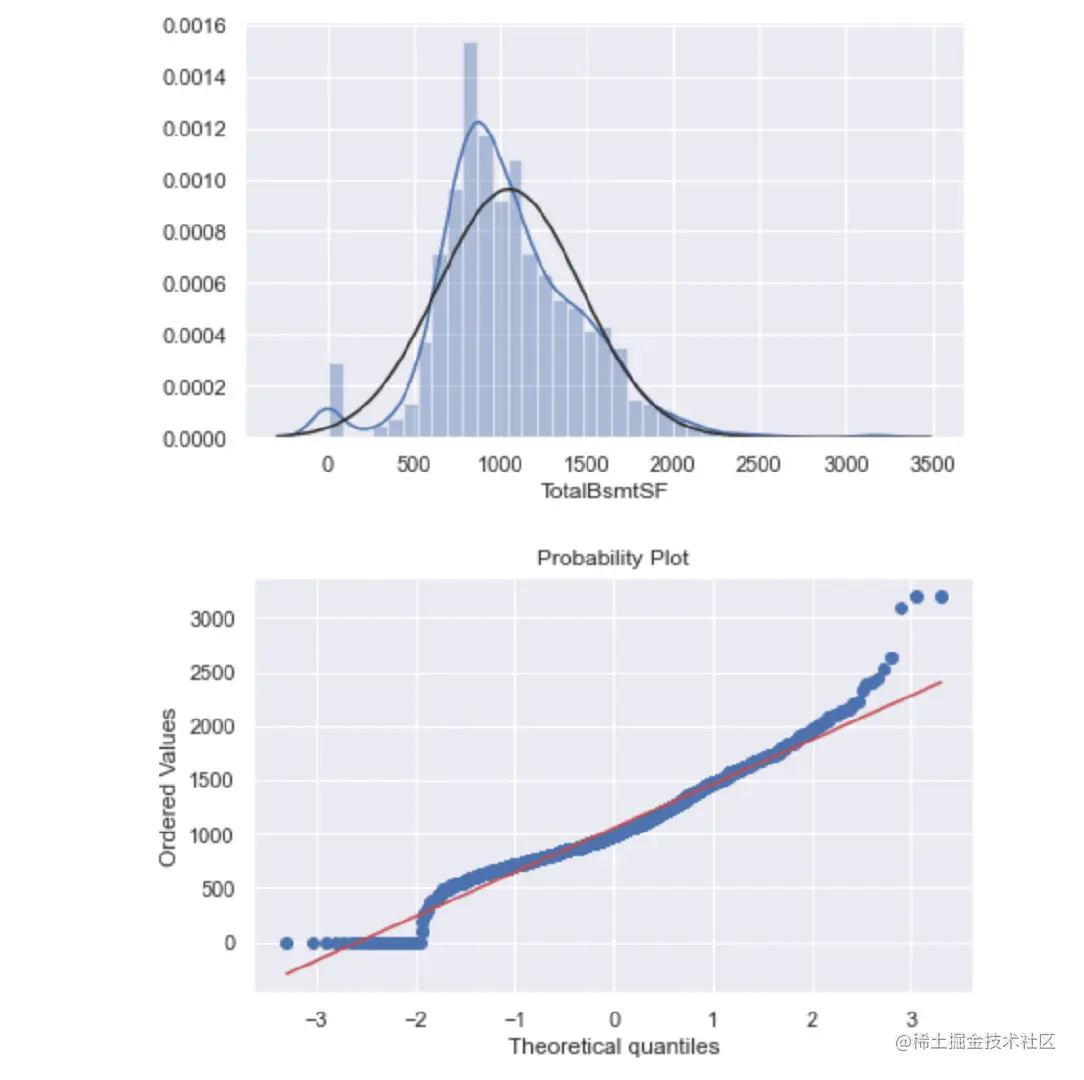How to deal with the special parts above ？

``````#  Add a column of data
train['HasBsmt'] = 0

#  When TotalBsmtSF>0  Then assign 1
train.loc[train['TotalBsmtSF']>0,'HasBsmt'] = 1

#  Logarithmic transformation ： be equal to 1 Part of
train.loc[train['HasBsmt']==1,'TotalBsmtSF'] = np.log(train['TotalBsmtSF'])

#  mapping
data = train[train['TotalBsmtSF']>0]['TotalBsmtSF']
sns.distplot(data,fit=norm)
fig = plt.figure()
res = stats.probplot(data, plot=plt)
Copy code ``````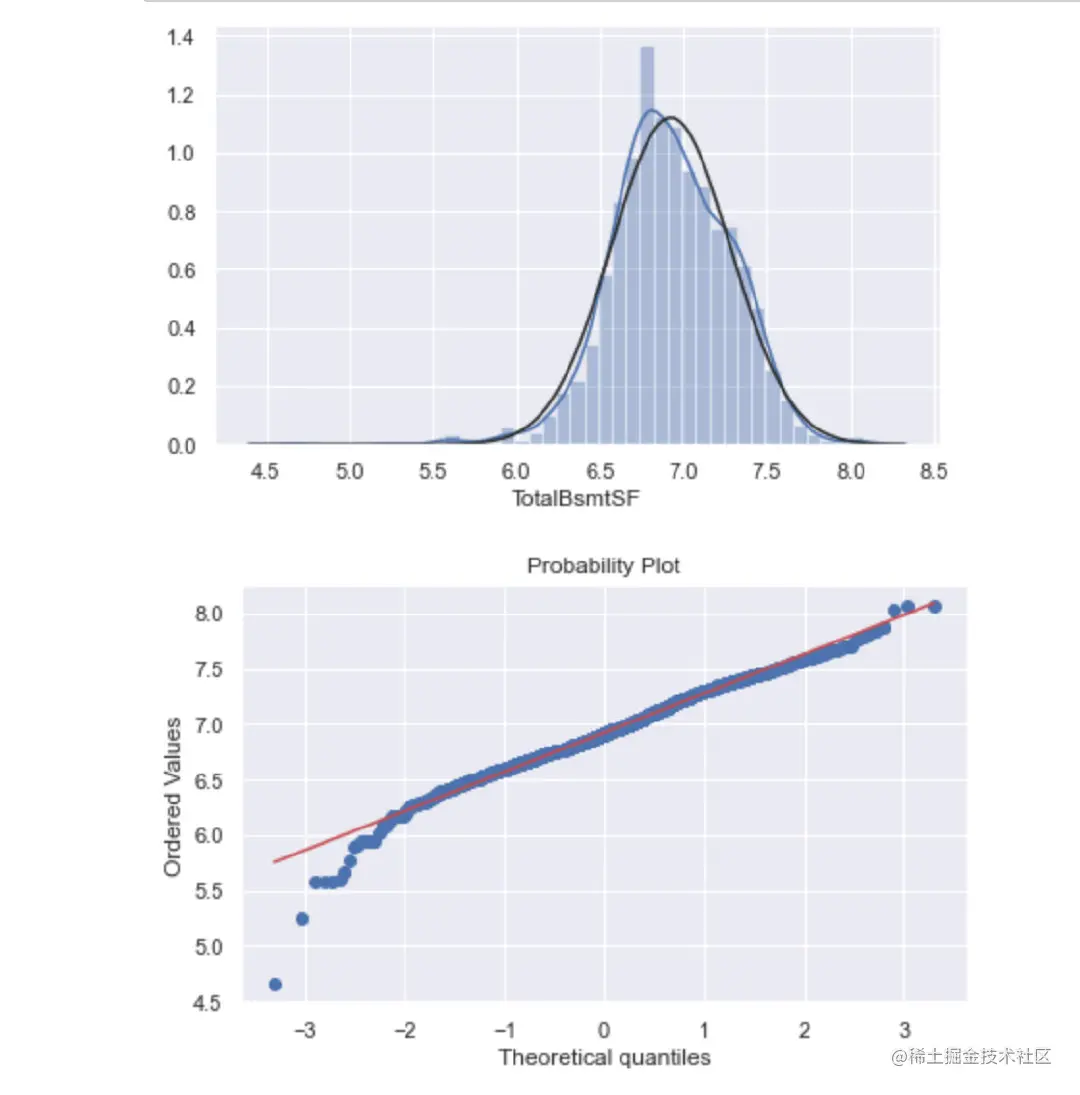## homoscedasticity

The best way to test the same variance between two variables is to plot .

The best approach to test homoscedasticity for two metric variables is graphically

1、 Discuss ：'SalePrice' and 'GrLivArea' The relationship between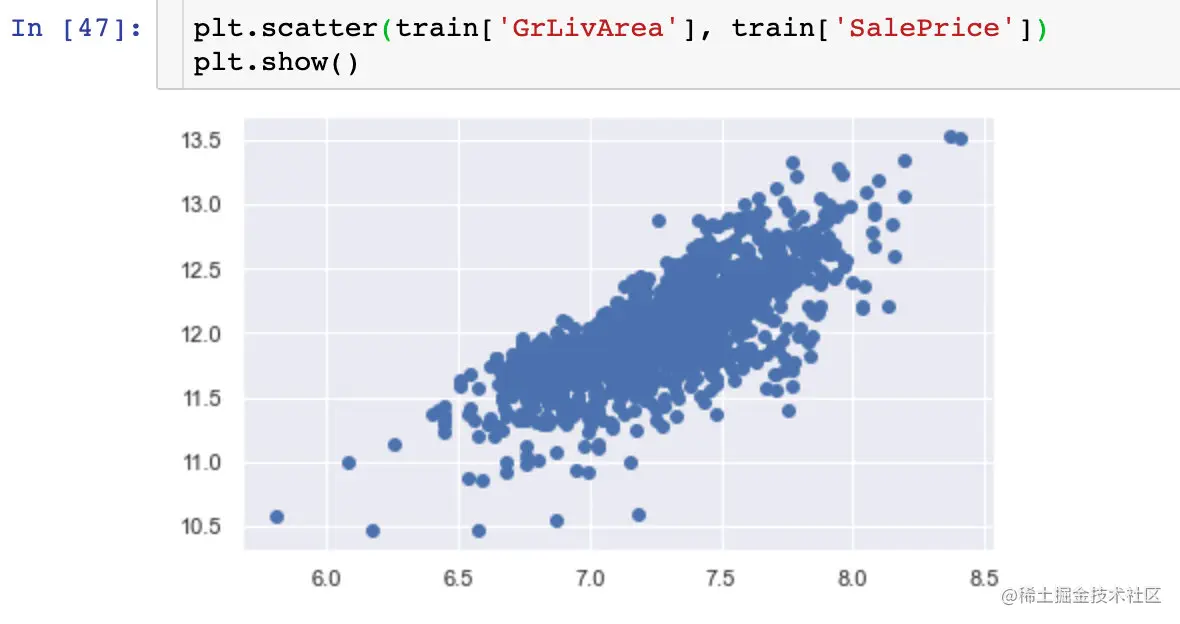2、 Discuss SalePrice' and 'TotalBsmtSF'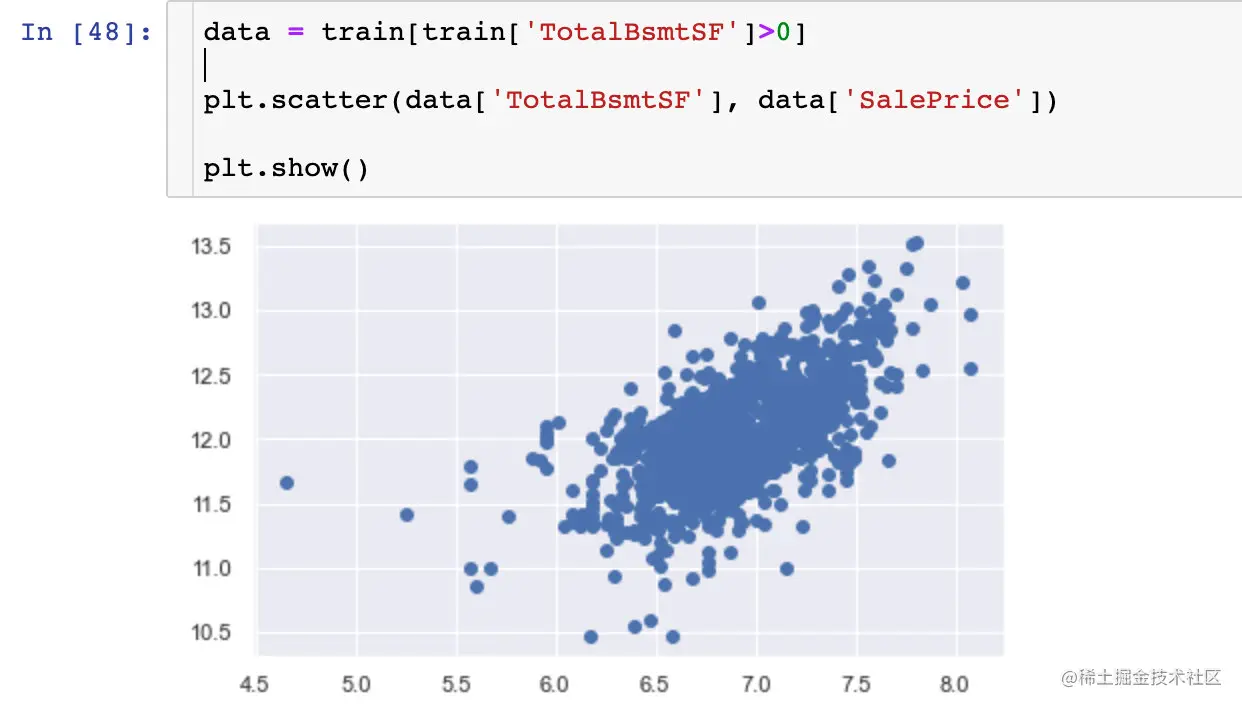We can say that, in general, 'SalePrice' exhibit equal levels of variance across the range of 'TotalBsmtSF'. Cool!

From the two pictures above , We see ： There is a positive relationship between sales price and the other two variables

## Generate dummy variables

Dummy variable ( Dummy Variables) Also known as dummy variable 、 Nominal variable or dummy variable , An artificial variable used to reflect qualitative attributes , Is a quantified independent variable , Usually the value is 0 or 1.

Pandas Medium get_dummies Functions can implement ：

``````train = pd.get_dummies(train)  #  Generate dummy variables
train
Copy code ``````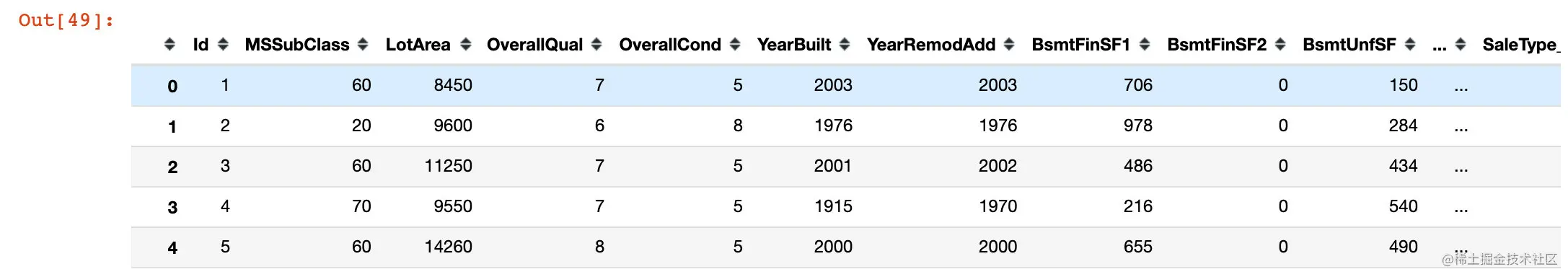## summary

thus , We have completed the following ：

1. Correlation analysis between overall variables
2. The variables are mainly analyzed “SalePrice”
3. Handle missing and outliers （ outliers ）
4. Did some statistical analysis , Change the classification variable into a dummy variable

I need to add some points for further study ：

• Multivariate statistical analysis
• Skewness and kurtosis
• The depth of dummy variables
• Standardization and normalization

About collection of data sets , Official account back office reply ： housing price . Look at the analysis process of the whole article , How do you feel ？